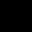# A started at 9.00 am with 6 mph and B started at 9.30 am with 8mph in the same direction. At what time they will meet?

## 3 Answers on this Question

1.Admin says:

Let they meet at a distance of x miles
time taken by A = x/6 and by B – x/8
x/6 â€“ x/8 = 1/2 (30 min = 1/2 hr)
4x â€“ 3x = 12
x = 12 miles
time taken by A = 12/6 = 2 hr.
meet at 9:00+ 2 hr = 11:00 am

2.Admin says:

11.00 AM

3.DK BOSS says:

A’s speed=6 mph ,B’s speed=8 mph
Let, after x hrs, they will meet.
so, the distance traveled by A in x hrs should be the same as the distance traveled by B in (x-1/2)hrs [as B started the journey after 30 min of A]
Thus, 6x=8(x-1/2)[as distance=speed*time]
=>8x-6x=4
=>2x=4
=>x=2
after 2 hrs they will meet so time=(9+2)=11.00 a.m

Scroll to top